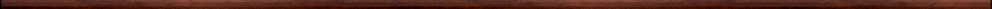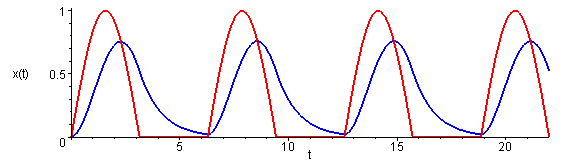Maple worksheets on Laplace transformsMathematical transforms:

They are all compatible with Classic Worksheet Maple 10.Integration prerequisites for Laplace transforms - intparts.mws

• Integration by parts
• Improper integrals

The Laplace transform - laplace.mws

• Introduction to the Laplace transform
• Laplace transforms of some standard functions
• Linearity of the Laplace transform operator

The inverse Laplace transform - invlaplace.mws

• The inverse Laplace transform operator.
• Some examples of inverse Laplace transforms.
• Provisional table of inverse Laplace transforms.

The Laplace transform method for solving some first order DE's - lapDE.mws

• The differentiation formula: L[f '(t)] = s L[f(t)] - f(0).
• Examples to illustrate the differentiation formula.
• Examples of solving 1st order DE's by the Laplace transform method.

Using partial fractions to find inverse Laplace transform - invlaplace2.mws

• Partial fractions and inverse Laplace transforms.
• Examples.
• Examples of solving 1st order DE's by the Laplace transform method.
• Partial fraction expansions involving irreducible quadratic factors in the denominator.
• More examples of solving 1st order DE's by the Laplace transform method.

The shift formula for the Laplace Transform - shift.mws

• The shift formula: If L[f(t)] = F(s), then L[exp(a t) f(t)] = F(s - a).
• Inverse Laplace transforms using the shift formula.
• Partial fraction expansions for the case of repeated factors in the denominator.
• More examples of solving 1st order DE's by the Laplace transform method.

Solving 2nd order DE's by the Laplace transform method - lapDE2.mws

• Extension of the differentiation formula for the Laplace transform operator.
• Examples of solving 2nd order DE's by the Laplace transform method.

More formulas for Laplace Transforms - morelap.mws

• An integration formula for the inverse Laplace transform operator.
• A 2nd differentiation formula for the Laplace transform.
• A 2nd integration formula for the Laplace transform.
• More standard Laplace transforms.
• Some standard inverse Laplace transforms.

Laplace transforms of piecewise functions - piecewise.mws

• The unit step function H(t-a) and its Laplace transform.
• The second shift formula: L[H(t-a) f(t)] = exp(-a s) L[f(t+a)].
• Examples of finding Laplace transforms using the 2nd shift formula.
• Finding Laplace transforms of piecewise functions.
• Inverse version of the second shift formula.

Differential equations involving piecewise functions - lapDE3.mws

• Differential equations arising from L-R and R-C series circuits
• Examples of differential equations involving piecewise functions

Laplace transforms of piecewise periodic functions - periodic.mws

• Periodic functions
• General method for finding Laplace transforms of periodic functions.
• Examples of Laplace transforms of piecewise periodic functions:
• Laplace transform of a square wave
• Laplace transform of a sawtooth wave
• Laplace transform of a triangular wave
• Laplace transform of the full-wave rectification of a sine wave
• Laplace transform of the half-wave rectification of a sine wave
• Laplace transform of a parabolic wave

Differential equations involving piecewise periodic functions - lapDE4.mws

• Laplace transforms of periodic functions as infinite sums.
• Differential equations with a piecewise periodic "forcing function":
• A first order DE with a square-wave "forcing function"
• A first order DE with a half-wave rectified sine wave "forcing function"
• A first order DE with a triangular wave "forcing function"

Another differential equation involving a piecewise periodic function - lapDE5.mws

• The Laplace transform of an alternating triangular wave.
• A 2nd order differential equation with an alternating triangular wave "forcing function".

Yet another differential equation involving a piecewise periodic function - lapDE6.mws

• The Laplace transform of an alternating trapezoidal wave.
• 2nd order differential equations with an alternating trapezoidal wave "forcing function".

A procedure for solving some 1st order differential equations by the Laplace transform method  - desolveLP.mws

• A procedure for solving first order DE's by using Laplace transforms - desolveLP.
• Examples

A procedure for solving some 2nd order differential equations by the Laplace transform method  - desolveL2.mws

• A procedure for solving 2nd order DE's by using Laplace transforms - desolveL2.
• Examples

Procedures for differential equations - DEsol.zipTop of page

Main index